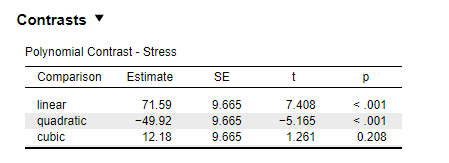#### Howdy, Stranger!

It looks like you're new here. If you want to get involved, click one of these buttons!

Supported by

# Divergence between JASP and SPSS ouptuts when computing polynomial contrasts for within-sjts designs

edited February 2019

Hi,
I am trying to reproduce David Howell's SPSS analysis of the aiport dataset (attached) in JASP. Dave's analysis is available at https://www.uvm.edu/~dhowell/StatPages/More_Stuff/RepMeasMultComp/RepMeasMultComp.html. When performing the polynomial contrast analysis of the effect of time on stress level (condition near aiport only), SPSS returns F(1, 99) = 1.721 p = .193 for the cubic trend while JASP returns t = 1.261 p = .208:Obviously the t statistic returned by JASP is not equal to the square root of F returned by SPSS. Could you please clarify why?
Best,
Mat

• Hi Mat,
I just ran the analysis in R to check those results. Here is the code I wrote:

``````myDat <- read.csv("/home/johnny/Downloads/airport.csv")

# Take subset for "Near" condition
myDat <- subset(myDat, LOCATION == 1)

# Turn data frame into the "long data" format
myDat <- data.frame(y = unname(unlist(myDat[,1:4])),
x = as.factor(rep(1:4, each = 100)),
ID = as.factor(rep(1:100, 4)))

# Set contrast
contrasts(myDat\$x) <- contr.poly(4)

# Run RM ANOVA
myAOV <- aov(y ~ x + Error(ID/x), data=myDat)

# extract relevant contrasts from AOV object